IF YOU CAN SEE THIS go to /sfa/Nuprl/Shared/Xindentation_hack_doc.html

### Substitution (second-order)

Substitution is used to give an Operator Definition in Nuprl, and showing how operators can be expanded according to definitions seems the clearest way to illustrate how substitution works.

First-order substitution is a method of inserting expressions into expressions, perhaps changing binding variables to avoid capture. For example according to the definition

Def {i...j} == {k: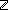i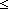k & kj }

the expression "{3...x+2}" expands to "{k:| 3k & kx+2 }", the expressions "3" and "x+2" being substituted for "i" and "j" in "{k:ik & kj }". But expression "{3...k+2}" expands to "{k@0:| 3k@0 & k@0k+2 }", avoiding a capture of "k".

Generally, one specifies a first order substitution by giving the "matrix", i.e. the expression into which one substitutes, and one gives a list of "target" variables to be replaced, and for each target one gives its "replacement expression". If we consider the replacement expressions as arguments, then the matrix and target variables together specify a "first-order K-place substitution function" if there are K targets given.

Second-order substitution is a method for inserting instances of a "pattern" into an expression, again changing binding variables as need be to avoid capture, where the pattern is itself simply instantiated by first order substitution. Consider the definition of symmetry for a relation.

Def Sym x,y:TE(x;y) ==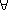a,b:TE(a;b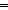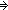E(b;a)

According to this definition, "Sym x,y:y<x+1" expands to "a,b:b<a+1a<b+1". It works like this. Of course, T is replaced by, but the "second-order" variable instances "E(a;b)" and "E(b;a)" are replaced by performing first-order substitution into "y<x+1". It is not necessary to use the same binding variables -- they will be changed as needed. Thus, "Sym u,v:v<u+1" also expands to "a,b:b<a+1a<b+1".

A second-order K-place variable takes expressions, K of them, as arguments in any given "instance" of that variable. In the above example, "E(?;?)" is a second-order 2-place variable, which is to say its instances are the expressions generated by filling in its 2 argument places with expressions. In the definition of symmetry there are three instances of this second-order variable, namely E(x;y), E(a;b) and E(b;a).

Generally, one specifies a second-order substitution by giving the matrix, a list of target variables which may now include second-order as well as first-order variables, and for each target variable, if it is a first-order variable then one gives its replacement expression, but if it is a second-order variable then one gives its "replacement function" which is a first-order substitution function (see above) with as many places as the second-order variable takes.

It is easy to see where the replacement functions for second order variables come from when expanding an operator instance according to an Operator Definition. The immediate subterms of a definiendum (left side) are taken as the target variables, and the subterms of the operator instance, in those places that have binding variables, are taken together with those binding variables to determine the replacement function (which is a first-order substitution function).

As a last example illustrating more complex substitution consider this definition:

Def Weird(xu,vF(u;v)) == F(F(x;0);x)

Weird(3; u,vv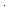v-u) expands to 33-(00-3)

Weird(3; v,uvv-u) expands to (33-0)(33-0)-3

Weird(3; u,vu) and Weird(3; v,uu) both expand to 3

Finally, observe that first-order variables can be construed simply as 0-place second-order variables, and then first-order substitution becomes a special case of second-order substitution.

(Feb 2001 - sfa )